# Dataintensive Computing Systems Query Optimization Costbased optimization Shivnath

• Slides: 41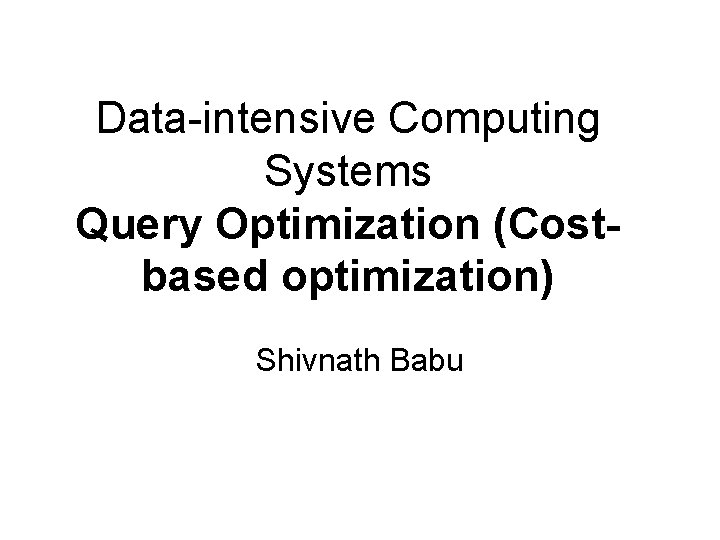Data-intensive Computing Systems Query Optimization (Costbased optimization) Shivnath Babu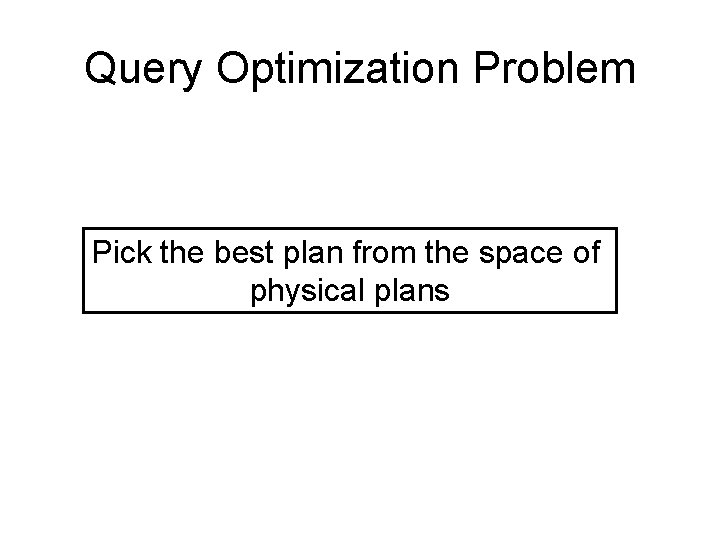Query Optimization Problem Pick the best plan from the space of physical plans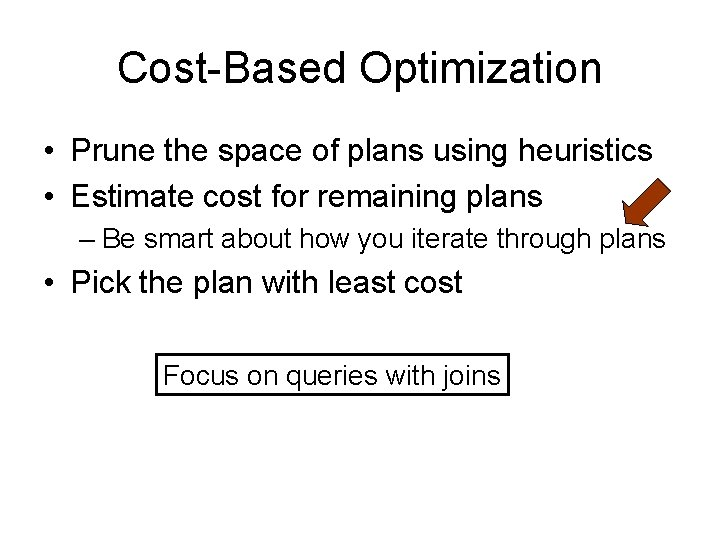Cost-Based Optimization • Prune the space of plans using heuristics • Estimate cost for remaining plans – Be smart about how you iterate through plans • Pick the plan with least cost Focus on queries with joins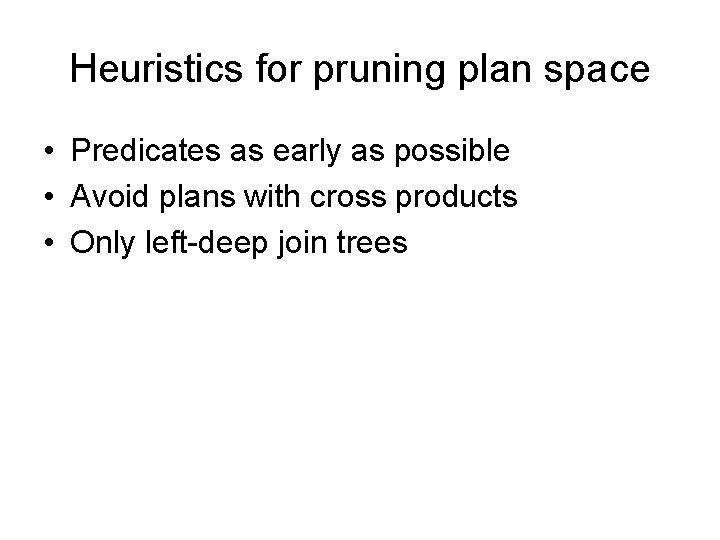Heuristics for pruning plan space • Predicates as early as possible • Avoid plans with cross products • Only left-deep join treesPhysical Plan Selection Logical Query Plan P 1 P 2 …. Pn Physical plans C 1 C 2 …. Cn Costs Pick minimum cost oneReview of Notation • T (R) : Number of tuples in R • B (R) : Number of blocks in RSimple Cost Model Cost (R S) = T(R) + T(S) All other operators have 0 cost Note: The simple cost model used for illustration only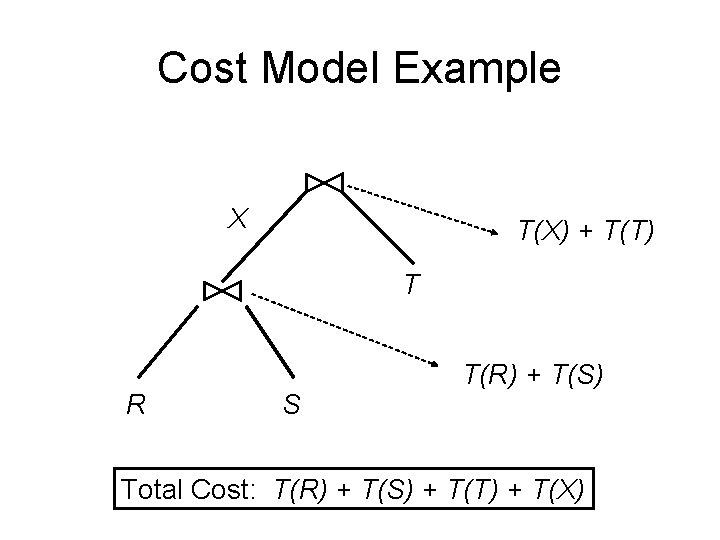Cost Model Example X T(X) + T(T) T R S T(R) + T(S) Total Cost: T(R) + T(S) + T(T) + T(X)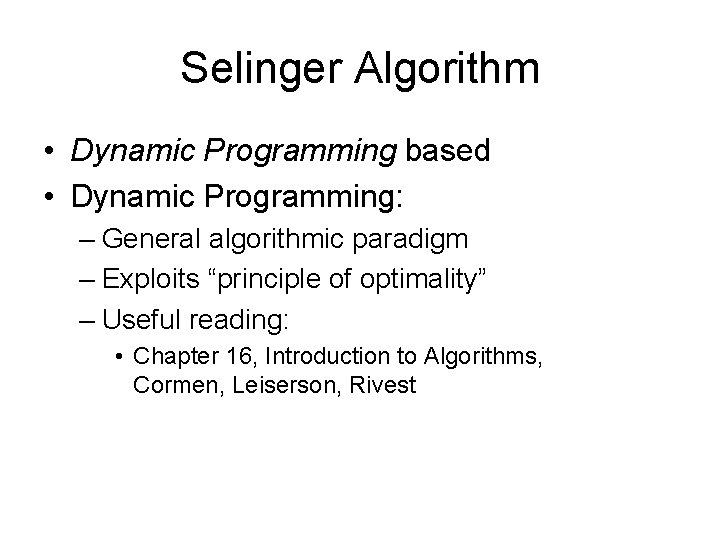Selinger Algorithm • Dynamic Programming based • Dynamic Programming: – General algorithmic paradigm – Exploits “principle of optimality” – Useful reading: • Chapter 16, Introduction to Algorithms, Cormen, Leiserson, RivestPrinciple of Optimality Optimal for “whole” made up from optimal for “parts”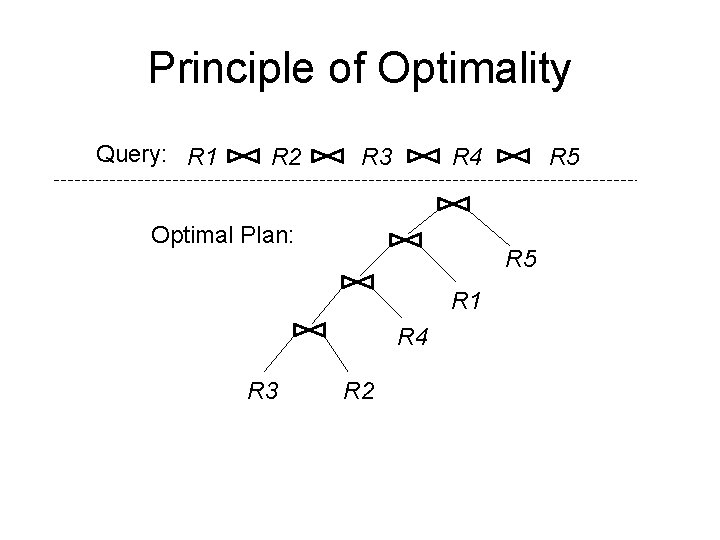Principle of Optimality Query: R 1 R 2 R 3 R 4 Optimal Plan: R 5 R 1 R 4 R 3 R 5 R 2Principle of Optimality Query: R 1 R 2 R 3 R 4 Optimal Plan: R 5 R 1 R 4 R 3 R 2 Optimal plan for joining R 3, R 2, R 4, R 1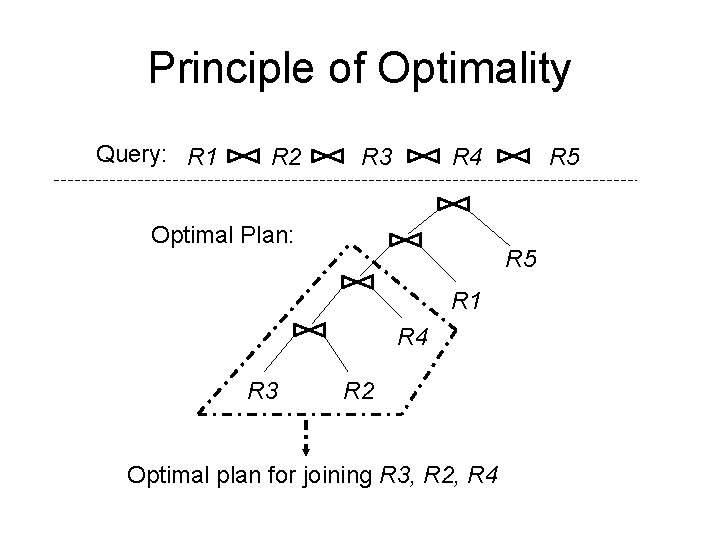Principle of Optimality Query: R 1 R 2 R 3 R 4 Optimal Plan: R 5 R 1 R 4 R 3 R 5 R 2 Optimal plan for joining R 3, R 2, R 4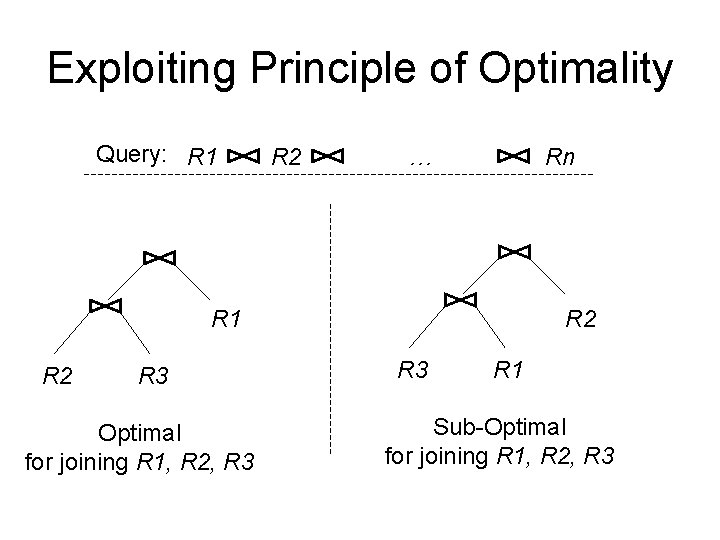Exploiting Principle of Optimality Query: R 1 R 2 … Rn R 1 R 2 R 3 Optimal for joining R 1, R 2, R 3 R 2 R 3 R 1 Sub-Optimal for joining R 1, R 2, R 3Exploiting Principle of Optimality Ri Rj R 2 R 3 Sub-Optimal for joining R 1, …, Rn R 1 A sub-optimal sub-plan cannot lead to an optimal planSelinger Algorithm: Query: R 1 R 2 R 3 Progress of algorithm { R 1, R 2, R 3, R 4 } { R 1, R 2, R 3 } { R 1, R 2, R 4 } { R 1, R 2 } { R 1, R 3 } { R 1, R 4 } { R 1 } { R 2 } R 4 { R 1, R 3, R 4 } { R 2, R 3 } { R 2, R 4 } { R 3, R 4 } { R 4 }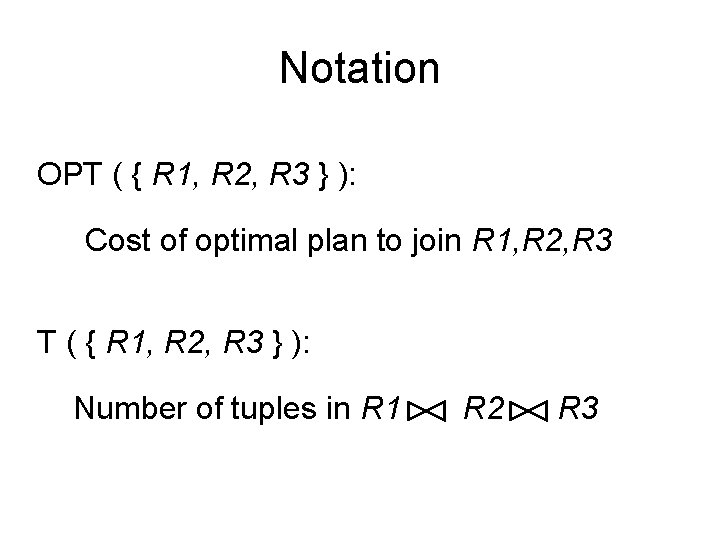Notation OPT ( { R 1, R 2, R 3 } ): Cost of optimal plan to join R 1, R 2, R 3 T ( { R 1, R 2, R 3 } ): Number of tuples in R 1 R 2 R 3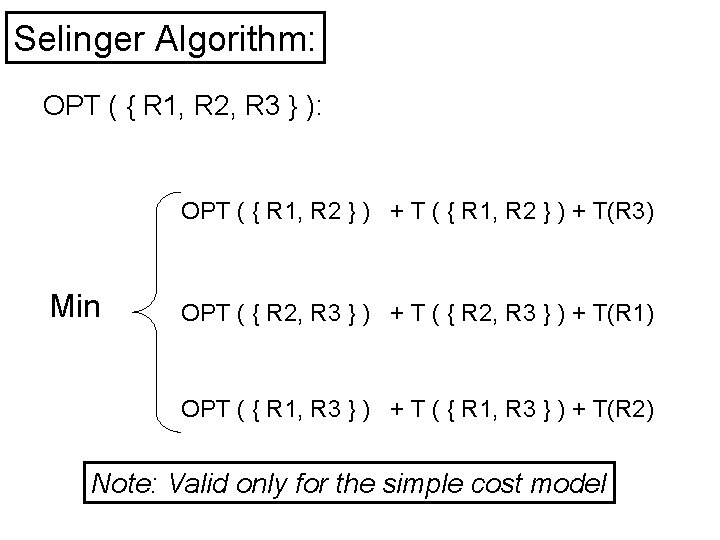Selinger Algorithm: OPT ( { R 1, R 2, R 3 } ): OPT ( { R 1, R 2 } ) + T(R 3) Min OPT ( { R 2, R 3 } ) + T(R 1) OPT ( { R 1, R 3 } ) + T(R 2) Note: Valid only for the simple cost model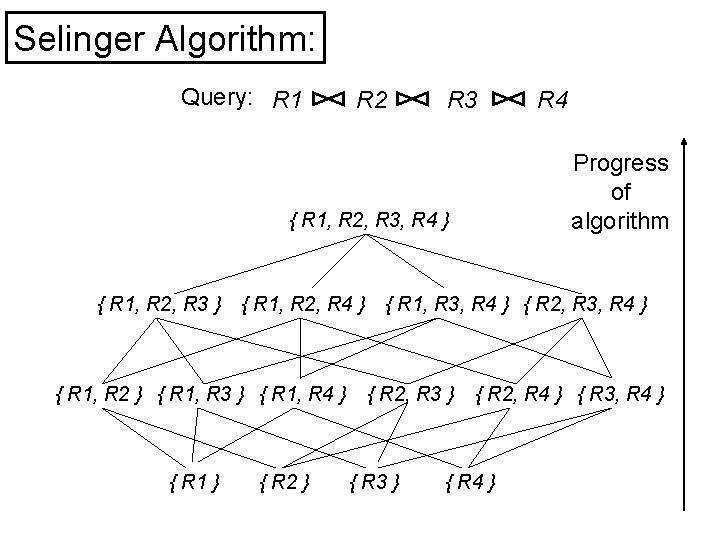Selinger Algorithm: Query: R 1 R 2 R 3 Progress of algorithm { R 1, R 2, R 3, R 4 } { R 1, R 2, R 3 } { R 1, R 2, R 4 } { R 1, R 2 } { R 1, R 3 } { R 1, R 4 } { R 1 } { R 2 } R 4 { R 1, R 3, R 4 } { R 2, R 3 } { R 2, R 4 } { R 3, R 4 } { R 4 }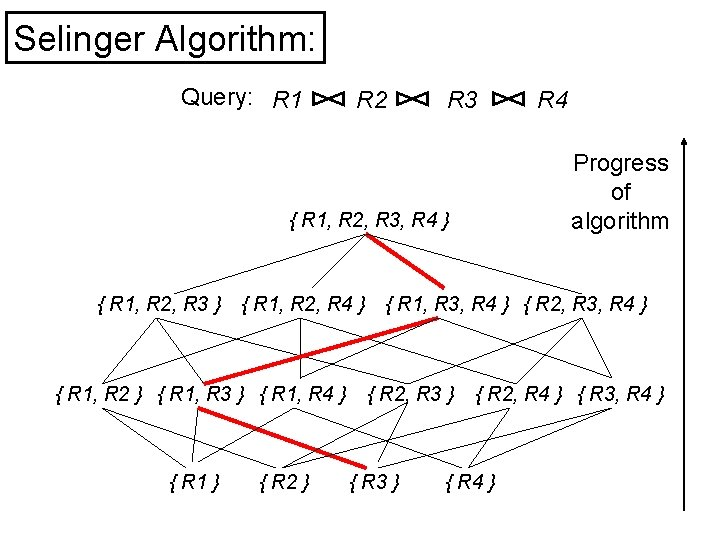Selinger Algorithm: Query: R 1 R 2 R 3 Progress of algorithm { R 1, R 2, R 3, R 4 } { R 1, R 2, R 3 } { R 1, R 2, R 4 } { R 1, R 2 } { R 1, R 3 } { R 1, R 4 } { R 1 } { R 2 } R 4 { R 1, R 3, R 4 } { R 2, R 3 } { R 2, R 4 } { R 3, R 4 } { R 4 }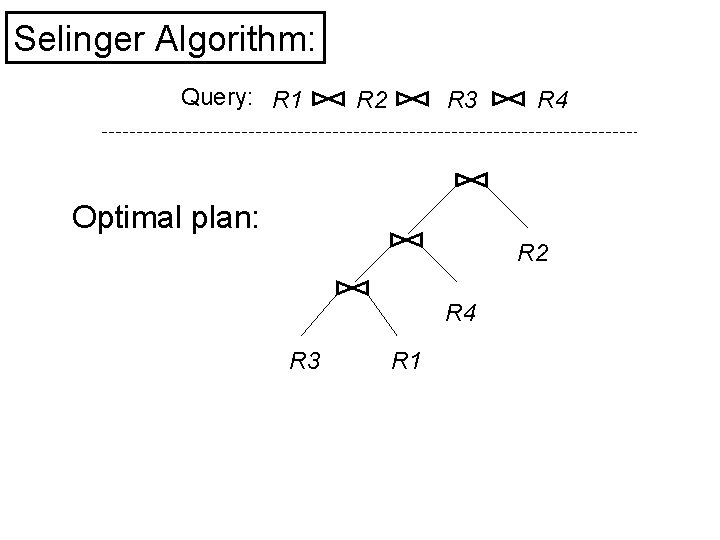Selinger Algorithm: Query: R 1 R 2 R 3 R 4 Optimal plan: R 2 R 4 R 3 R 1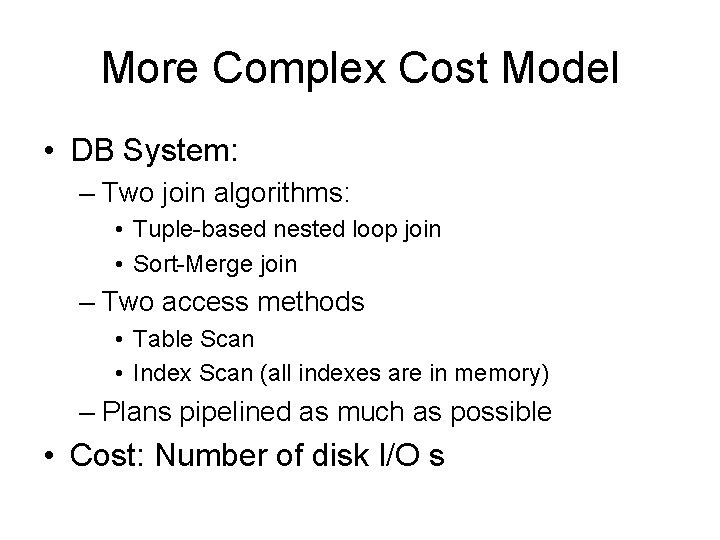More Complex Cost Model • DB System: – Two join algorithms: • Tuple-based nested loop join • Sort-Merge join – Two access methods • Table Scan • Index Scan (all indexes are in memory) – Plans pipelined as much as possible • Cost: Number of disk I/O s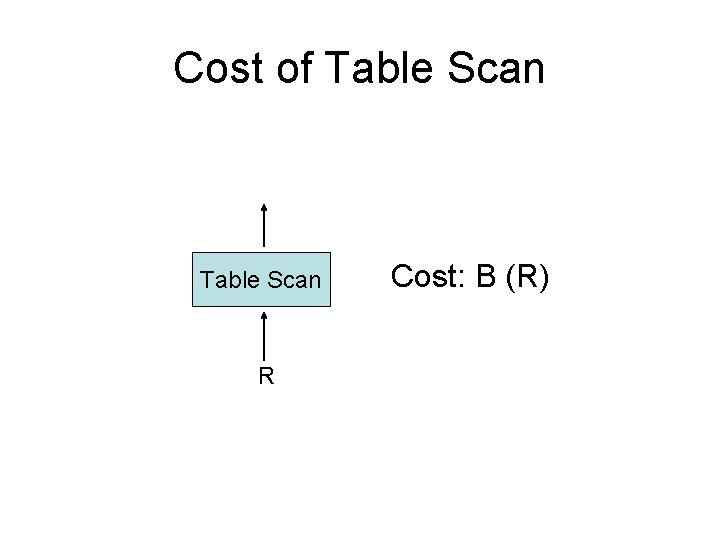Cost of Table Scan R Cost: B (R)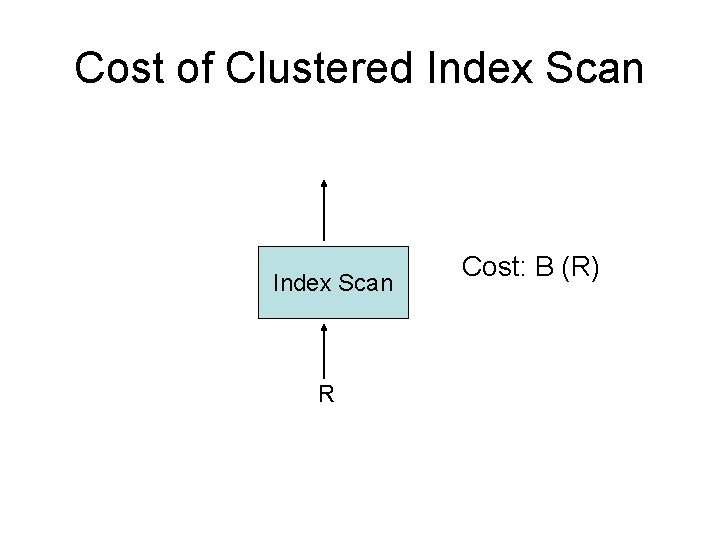Cost of Clustered Index Scan R Cost: B (R)Cost of Clustered Index Scan X R. A > 50 Index Scan R Cost: B (X)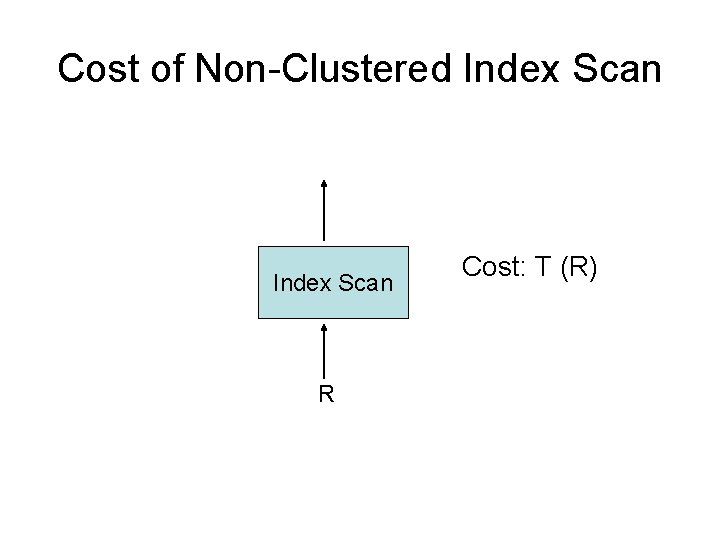Cost of Non-Clustered Index Scan R Cost: T (R)Cost of Non-Clustered Index Scan X R. A > 50 Index Scan R Cost: T (X)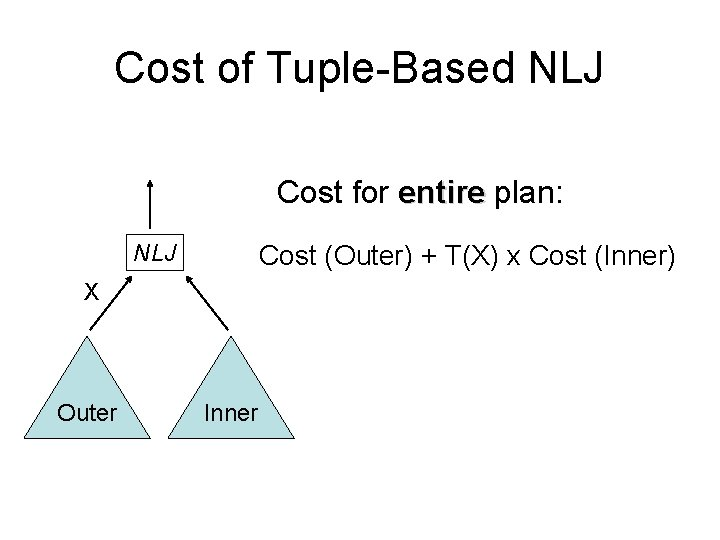Cost of Tuple-Based NLJ Cost for entire plan: NLJ Cost (Outer) + T(X) x Cost (Inner) X Outer Inner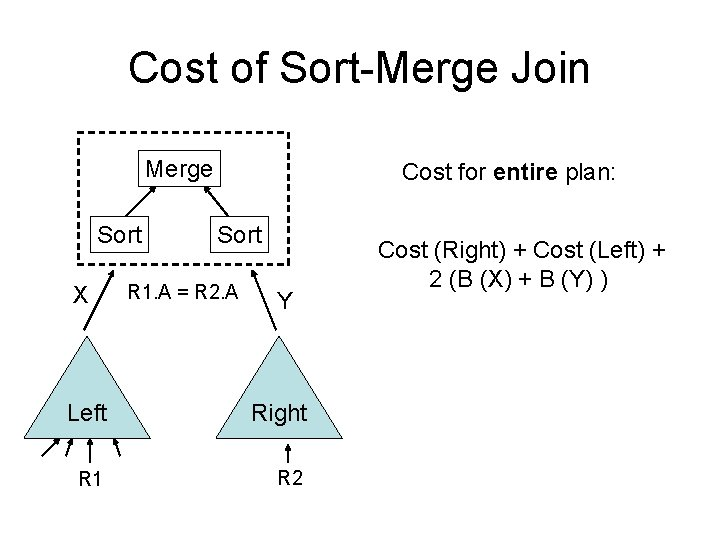Cost of Sort-Merge Join Merge Sort X Cost for entire plan: Sort R 1. A = R 2. A Y Left Right R 1 R 2 Cost (Right) + Cost (Left) + 2 (B (X) + B (Y) )Cost of Sort-Merge Join Merge Cost for entire plan: Sort X R 1. A = R 2. A Y Left Right R 1 R 2 Cost (Right) + Cost (Left) + 2 B (Y) Sorted on R 1. ACost of Sort-Merge Join Merge Cost for entire plan: Cost (Right) + Cost (Left) X R 1. A = R 2. A Y Sorted on R 2. A Left Right R 1 R 2 Sorted on R 1. ACost of Sort-Merge Join Bottom Line: Cost depends on sorted-ness of inputsPrinciple of Optimality? Query: R 1 Optimal plan: R 2 SMJ R 3 R 4 R 5 (R 1. A = R 2. A) Scan Plan X R 1 Is Plan X the optimal plan for joining R 2, R 3, R 4, R 5?Violation of Principle of Optimality (sorted on R 2. A) Plan X Suboptimal plan for joining R 2, R 3, R 4, R 5 (unsorted on R 2. A) Plan Y Optimal plan for joining R 2, R 3, R 4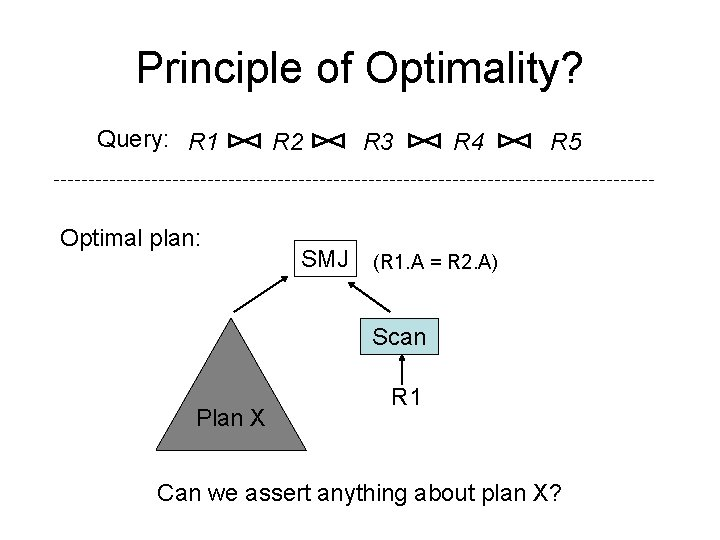Principle of Optimality? Query: R 1 Optimal plan: R 2 SMJ R 3 R 4 R 5 (R 1. A = R 2. A) Scan Plan X R 1 Can we assert anything about plan X?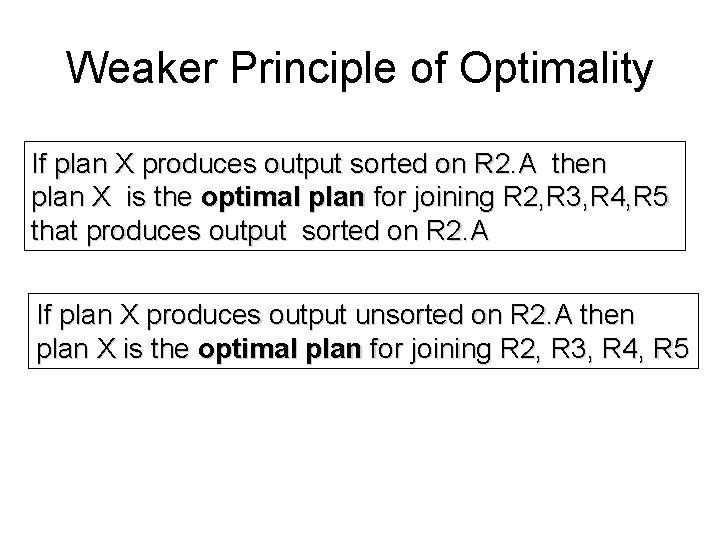Weaker Principle of Optimality If plan X produces output sorted on R 2. A then plan X is the optimal plan for joining R 2, R 3, R 4, R 5 that produces output sorted on R 2. A If plan X produces output unsorted on R 2. A then plan X is the optimal plan for joining R 2, R 3, R 4, R 5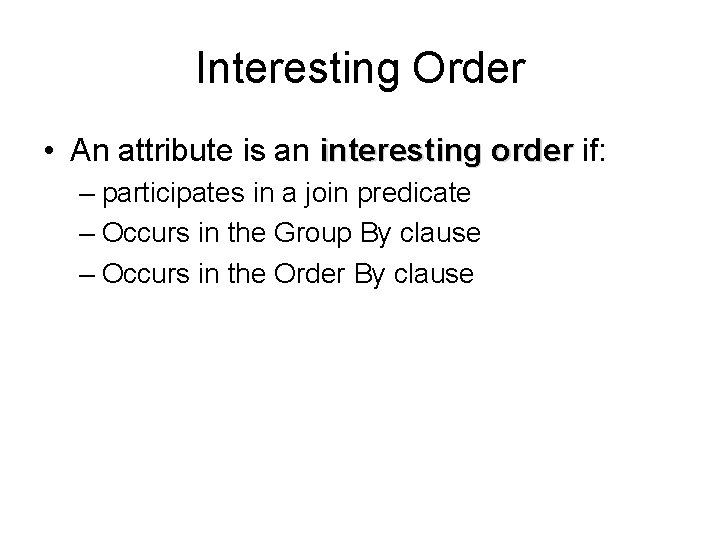Interesting Order • An attribute is an interesting order if: – participates in a join predicate – Occurs in the Group By clause – Occurs in the Order By clause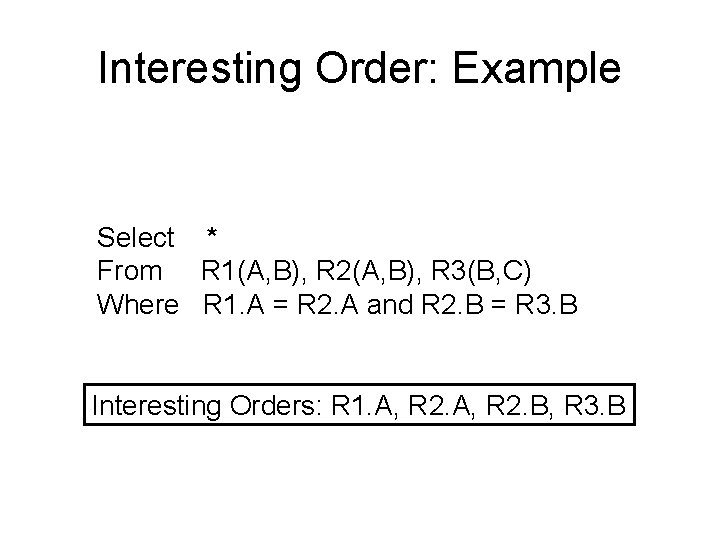Interesting Order: Example Select * From R 1(A, B), R 2(A, B), R 3(B, C) Where R 1. A = R 2. A and R 2. B = R 3. B Interesting Orders: R 1. A, R 2. B, R 3. B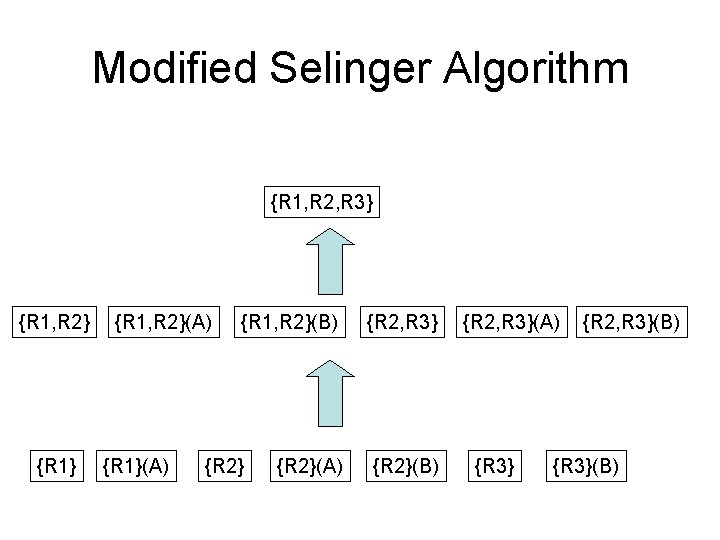Modified Selinger Algorithm {R 1, R 2, R 3} {R 1, R 2}(A) {R 1, R 2}(B) {R 2}(A) {R 2, R 3} {R 2}(B) {R 2, R 3}(A) {R 3} {R 2, R 3}(B) {R 3}(B)Notation {R 1, R 2} (C) Optimal way of joining R 1, R 2 so that output is sorted on attribute R 2. CModified Selinger Algorithm {R 1, R 2, R 3} {R 1, R 2}(A) {R 1, R 2}(B) {R 2}(A) {R 2, R 3} {R 2}(B) {R 2, R 3}(A) {R 3} {R 2, R 3}(B) {R 3}(B)